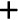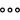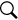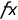# Creating Calculated Measures

You can use the Calculation Editor to create calculated measures.

## Prerequisites

A chart or table must be selected.

## Context

Calculated measures allow you to perform mathematical and Boolean operations on your data. For example, you can use a calculated measure to chart the effect a sales increase of 20% would have on profits.

Note
The number format chosen inProfile SettingsUser Preferencesinfluences the expected input format for Story Calculated Measures and Calculated Dimensions:
• Choosing a number format that uses periods (.) as decimal separators means that commas (,) must be used to separate function parameters (for example, IF(Condition, ValueIfConditionIsTrue, ValueIfConditionIsFalse)).

• Choosing a number format that uses commas (,) as decimal separators means that semi-colons (;) must be used to separate function parameters (for example, IF(Condition; ValueIfConditionIsTrue; ValueIfConditionIsFalse)).

If the formula is typed in from scratch, the correct function auto-completion happens based on the user preferences. However, if you copy and paste a full formula string, auto-complete won't be able to adapt if there is a mismatch between separators used and the user preferences.

The following functions, conditions, and operators are available for creating calculated measures. For descriptions of each option, see All Formulas and Calculations.

Functions Conditions Operators
IF() AND + (addition)
ABS() OR - (subtraction)
LOG() > (greater than) * (multiplication)
LOG10() < (less than) / (division)
INT() >= (greater than or equal)
FLOAT() <= (less than or equal)
DOUBLE() = (equal)
POWER() != (not equal)
LENGTH()
LIKE()
SUBSTRING()
ISNULL()
NOT()

## Procedure

1. Open the Calculation Editor using one of the following methods:
• Select a chart and do one of the following:

• In Builder, chooseAdd MeasureCalculationsClick to Create a New Calculation.

1. In Builder, beside Chart Structure, select(Add Chart Components), and then select Add Cross Calculations.

A Cross Calculations section is added with the default dimension Measure Values.

2. SelectSelect Cross CalculationClick to Create a New Cross Calculation.

• Select a table.

1. Under Rows or Columns in the Builder tab, select Add Measures/Dimensions and add either the Account or Cross Calculations dimension.

Tip
You can rename the Cross Calculations dimension.
2. For the row or column that you added in the Builder tab, selectAdd Calculation.
Note
The option to create a new calculation may not appear if calculations are not possible for the chart type or model.

The Calculation Editor appears.

2. Select Calculated Measure from the list.
3. Enter a name for the calculated measure.
4. Enter a formula in the Edit Formula area.

Existing input controls appear in the Available Objects list, and can be added to a formula.

Note
Only single value numeric input controls can be used in formulas.

You can add preset functions, conditions, and operators, by selecting options in the Formula Functions list. You can use IF conditional functions, and you can display a list of possible formulas for the function by pressing Ctrl + Space bar.

The formula editor supports auto-complete and the following shortcuts:
• [ – Returns all relevant measures:
• Adding a calculation to the Account dimension: shows Account dimension members.
• Adding a calculation to the Cross Calculations dimension: shows Cross Calculations dimension members.
• # – Returns all calculations (that is, measures created using the Calculation Editor).
• @ – Returns input controls. (Only single value numeric input controls are returned.)
Tip

In the list of measures, you see both the measure ID and the measure description. After you select a measure, the formula editor area shows only the measure ID. To view the measure description, click outside the formula editor area.

5. (Optional) Create a calculation input control.
Note
Calculation input controls can be created for charts only, not for tables.
1. Under INPUT CONTROLS, select Create New.
2. Enter a name for the input control.
3. Select Existing Dimension to allow users to pick from members of a dimension, or Static List to add custom values as options for the input control.
• Existing Dimension
1. Select a model, select a dimension, and then select Click to Add Values.

2. Select values from the list of available members.

If you select Exclude selected members, all members except the ones selected will be included in the input control. You can use(Search) to find specific values. When you expand the list beside the search icon, you can choose to view the member Description, ID and Description, or ID.

3. Expand the Settings for Users section, and then choose whether users can do the following in the input control: Single Selection, Multiple Selection, or Multiple Selection Hierarchy.

4. Select OK.

• Static List
1. Select Click to Add Valuesand choose either Select by Range or Select by Member.
Note

Choose Select by Range to specify a range for a numeric slider. Choose Select by Member for an input control based on a defined set of members.

The Select by Range option appears only if dimension values are numerical or date based. If the dimension is date based, you can also select quarter, month, or year from the slider that appears. Ranges can be fixed or dynamic; for example, you could choose the fixed range January 2017 to December 2017. If this story is opened in 2018, the story will still show 2017 data. For dynamic date ranges, in addition to the above granularities, these granularities are also available: current year, current quarter, and current month. For more information, see Story and Page Filters.

2. To create a numeric slider, enter the Min and Max values for your range in the Set Values for Custom Range dialog. You can optionally set an Increment value for the slider.

3. Select OK.

4. To create a member based input control, add numeric values to the Custom Members area in the Select Values from Custom LOV dialog, and then select Update Selected Members.

5. Expand the Settings for Users section, and then choose whether users can do the following in the input control: Single Selection, or Multiple Selection.

6. Select OK.

4. Select OK.

The input control you created appears under Available Objects.

5. Select the input control to add it to a formula.
6. Select Format to check if your formula is valid.
7. Select OK.

## Results

A measure is created based on the formula you entered. In a table, it is added as a new member of the Account or Calculations dimension. In a chart, it is displayed as a new measure or a new cross calculation dimension.

If the formula uses an input control, it will appear on the canvas.

On the canvas, input controls are indicated by the(Formula) icon. If you hover over the icon, all calculations associated with the input control are displayed. By default, the input control is displayed in token mode where input values can be selected from a drop-down list. The input control can be expanded into widget mode, where radio buttons appear beside each value.